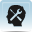# Resource 4: Summary of forces for the teacherBackground information / subject knowledge for teacher

 Forces are pushes and pulls.A force has two characteristics: size and direction.When forces act, they can change the shape of the things they act on. There is a bigger change from a bigger force.We can use the change to provide a quantitative measure of forces.Forces can be measured using a spring balance or force meter (newtonmeter) in units called newtons.An object’s weight is the force of gravity acting on it, thus weight is a force.The mass of an object is a measure of the amount of matter in it. It is constant and does not change.All objects exert a force on each other: the size of the force depends upon their masses.Gravity is the force that holds you down to the planet Earth.The force of gravity is smaller on the moon than on Earth because there is less matter in the moon (smaller mass) than in the Earth.On the Earth, every kilogram is pulled by gravity with a force of around 10 newtons towards the centre of the Earth.There is a distinction between mass and weight. Mass is an intrinsic property of an object – no matter where you are, you always have the same mass. Your weight is the gravitational force that acts on you. Your weight acts downwards, towards the centre of the Earth.Mass and weight have different units. Mass is measured in grams and kilograms, whereas weight (a force) is measured in newtons.Friction is a force that resists movement.A force is needed to start something moving, speed it up, slow it down or change its direction.The bigger the force, the greater the acceleration (or deceleration) on a fixed mass. When no overall force is acting on an object, it will remain stationary or continue at a steady speed in a straight line.

Adapted from: Developing Subject Knowledge – Jane Devereux

Resource 3: Force word spiders or burrs

Resource 5: How can we reduce friction?